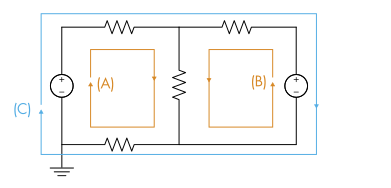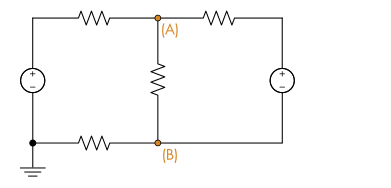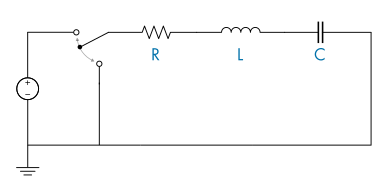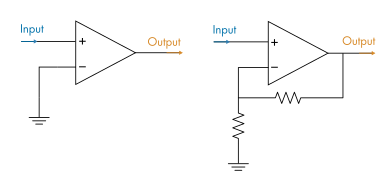## DC Circuit Analysis

Version 1.0.3 (5.99 MB) by
Virtual labs for DC circuit analysis.

Updated 17 May 2022

From GitHub

# DC Circuit AnalysisCurriculum Module
Created with R2021b. Compatible with R2021b and later releases.

## Description

This curriculum module teaches DC circuit analysis using interactive live scripts and Simscape™ models. Essential topics such as mesh analysis, RLC circuits, and op amps (among others) are discussed. The materials contain two components for each topic: an introduction and a virtual lab. The introduction explains the technique and offers opportunities to develop intuition using simulation. In the labs, students apply their knowledge to analyze circuits, take measurements, and design circuits to meet requirements. Throughout the module, virtual analogs to standard instruments are used to familiarize students with standard measurement procedures. In the penultimate module, students measure the transient responses of overdamped, underdamped, and critically damped RLC circuits using a virtual signal generator and oscilloscope.

Get started with the DC Circuit Analysis curriculum module by downloading and unzipping the repository. Then, double-click the project .prj file inside MATLAB®. From there, you can follow the landing page instructions to get started with the examples and labs.

## Details

Module Learning Goals
1. Mesh Analysis• Use mesh analysis to compute currents through a linear circuit.
• Solve the matrix form of circuit equations in MATLAB.
• Model and analyze linear circuits in Simscape.
2. Nodal Analysis• Use nodal analysis to compute voltages.
• Solve the matrix form of circuit equations in MATLAB.
• Model and analyze linear circuits in Simscape.
3. Thevenin and Norton Equivalent Circuits• Explain Thevenin and Norton equivalent circuits.
• Solve for Thevenin and Norton equivalents.
• Compare circuits to their Thevenin and Norton equivalents.
4. RC Circuits• Simulate charging and discharging RC circuits.
• Plot solutions of RC circuit differential equations.
• Measure the time constant of an RC circuit.
5. RLC Circuits• Compare the response of first and second order circuits.
• Analyze a second order RLC circuit differential equation.
• Solve for the natural frequency and damping in an RLC circuit.
• Compare underdamped, overdamped, and critically damped circuits.
6. Op Amps• Describe the open-loop behavior of an op amp.
• Compare ideal and non-ideal op amp behavior.
• Analyze negative feedback op amp circuits.
• Design circuits using combinations of basic op amp circuits.

## How-to Videos

These short videos demonstrate some of the basic techniques used to simulate and measure circuits in the module.

SimulateCircuit.mp4
VirtualMultimeter.mp4
VirtualOscilloscope.mp4

Note: The videos can be viewed from the GitHub repository readme.

## Suggested Prework

MATLAB Onramp – a free two-hour introductory tutorial that teaches the essentials of MATLAB.

Circuit Simulation Onramp – a free two-hour introductory tutorial that teaches the basics of simulating electrical circuits in Simscape.

## Products

MATLAB, Simulink®, Simscape, Simscape Electrical™, DSP System Toolbox™

The license for this module is available in the LICENSE.md file in this GitHub repository.

## Educator Resources

The lab solutions are available upon instructor request. If you would like to request solutions or have a question, contact the MathWorks online teaching team.

### Cite As

Chad Allie (2023). DC Circuit Analysis (https://github.com/MathWorks-Teaching-Resources/DC-Circuit-Analysis/releases/tag/v1.0.3), GitHub. Retrieved .

##### MATLAB Release Compatibility
Created with R2021b
Compatible with R2021b and later releases
##### Platform Compatibility
Windows macOS Linux
##### Communities
More Files in the  Distance Learning Community

### Community Treasure Hunt

Find the treasures in MATLAB Central and discover how the community can help you!

Start Hunting!

#### VirtualLabs

Version Published Release Notes
1.0.3

See release notes for this release on GitHub: https://github.com/MathWorks-Teaching-Resources/DC-Circuit-Analysis/releases/tag/v1.0.3

1.0.2

See release notes for this release on GitHub: https://github.com/MathWorks-Teaching-Resources/DC-Circuit-Analysis/releases/tag/v1.0.2

1.0.1

See release notes for this release on GitHub: https://github.com/MathWorks-Teaching-Resources/DC-Circuit-Analysis/releases/tag/v1.0.1

1.0.0

To view or report issues in this GitHub add-on, visit the GitHub Repository.
To view or report issues in this GitHub add-on, visit the GitHub Repository.